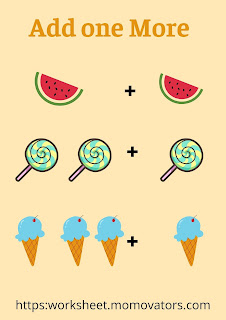-->

# How to teach addition for class 1

Confused about how to start teaching addition? You will find the 7 different ideas and step-by-step methods to teach the addition to grade 1 children. The addition is the very first mathematical calculation concept to learn. So, the child might feel difficult to understand the addition concept. The child must be mastered in counting before learning the addition concept. Read on the below post for helping the upper kindergarten (UKG) and grade 1 children learn how to add.TEACH ADDITION TO CLASS 1

I have shared the ideas and strategies which I have followed to teach the addition to my child. I started teaching the addition concept when my little one was 5 years old. I hope these ideas would assist you in teaching the addition to children.

#### 1. One More Concept

It is the first and foremost step in teaching the addition to the beginners. It is one of the simplest forms of addition. The child might be aware of the counting. The child can easily understand the concept of addition with this simple method. You can teach this concept with manipulatives or toys.

You can say to them that there are 2 apples and when you add one more it becomes 3 apples. The children can easily do this with counting. The child would count and say the number. The children can understand that there is an increase in number or quantity. You should use the terms such as together, total, altogether while teaching the addition through one more concept.ADDITION CLASS 1

#### 2. Using manipulatives

When the child is mastered in adding using the One More concept. The next step is to teach the addition using the manipulatives. The child can easily learn any mathematical concepts with the manipulatives. They can learn the concepts of addition visually through manipulatives. You can use the building blocks, lego toys, or candies to teach the addition concept visually.

Always start teaching the new concepts with the minimum numbers so that the child can understand easily. You can keep 3 blue colors building blocks and 2 red colors building blocks. Ask them "how many blocks are there altogether?".  So, that the child can add and say by counting the number of objects.

#### 3. Provide the explicit teaching

When the child understands the concept of addition. The next step is to teach the addition signs and terms. Now, the child is ready to write it in an equation. They can write the addition in a sentence. You can draw a picture or lines below the numbers and make them do addition.

You need to use the words such as add, join, more, sum, equal, together, and all together. When you use these addition terminologies they will aware of it. It would be helpful for them when they do the addition word problem. Refer to the below image for teaching the addition by writing it in a sentence.ADDITION WITH PICTURES

Eg:  1 + 3 = 4; You can draw the lines or pictures below the numbers so that the child might feel easy to do this. Once they are mastered in doing it, You no need to draw the pictures or lines.

#### 4. Teach addition using the number line method

Draw the number line from 0 to 10. The child needs to jump to the first number and then from the first number to the second number. The number line method helps the child to understand forward counting and increase in numbers.

#### 5. Addition through Finger Method

By this time, the child would have been in mastered addition equations and terminology. Now, the child can do addition using the finger method. Most of the schools would follow this method to teach addition to the class 1 children.

In the finger method, the child can keep the first number in the left hand and the second number in the right hand. For example; adding 3 + 4; 3 on the left hand and 4 on the right hand.

#### 6. Mind method

The mind method is introduced after the grade 1 child is mastered in doing addition using their fingers. You can teach addition to children using the mind method. You can teach the child to keep the bigger number in mind and the smaller number in hand. The child needs to do forward counting from the bigger number.

#### 7. Skip counting

Skip counting is the last step. The child can learn to do addition faster with the concept of skip counting. You can teach the double addition such as 2, 4, 6, and so on using the skip counting concept.

### Final Note

When you teach addition with the fun activities and worksheets, then the child would learn and understand the concepts easily. I hope this post on "How to teach addition to grade 1?" would give you some ideas on teaching addition to class 1 children.### This post have 0 Comment

Next article Next Post
Previous article Previous Post